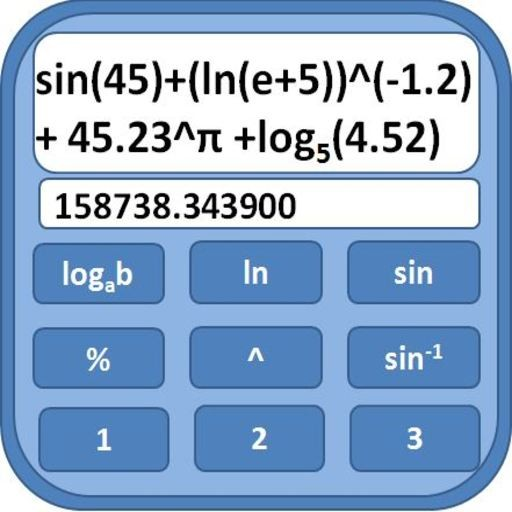## FX-991MS Scientific Calculator

### by Mohammed ujjainwala

Free
2322
Categories Tags
###### Description

Features:
1) Easy to edit large expressions. Just click anywhere on clipboard and edit your expression.
2) It can also show number as big as factorial of 5000. Just scroll down to see such big numbers.
3) No need to press shift. Just scroll down to use more functions.
4) You can write expressions with 500 operands.
5) You can use Infinite Parentheses

Functions:
1) Basic Math Operations such as divide,add,subtract,multiply,modulo,factorial etc
2) Can calculate nth root of a number
3) Can calculate factorial up to 5000
4) Can Evaluate log with base between 1-100
5) Trigonometric Functions
6) Number Base Conversion from 2 to 16
7) Matrix operations
8) You can perform operations on large numbers
9) Can Calculate exponent with decimal power

Note :
ln(23)^2 will be evaluated as ln(23^2) i.e. first 23^2 will evaluated which is 529 then ln(529) will be evaluated. If you want ln(23) should get evaluated first then write (ln(23))^2. Please use parentheses to get proper result otherwise you will get unexpected result.

This scientific calculator is made to make editing of large expressions easy. I have faced situation in my engineering where I have to write large expressions with little changes again and again. So I made this scientific calculator where you can easily edit and write large expressions. One more great feature of this scientific calculator is that you can deal with large numbers i.e. you can even calculate Factorial of 5000. One more thing you don't need to press shift this will save lot of time.

• AOTD

1581

4975

897